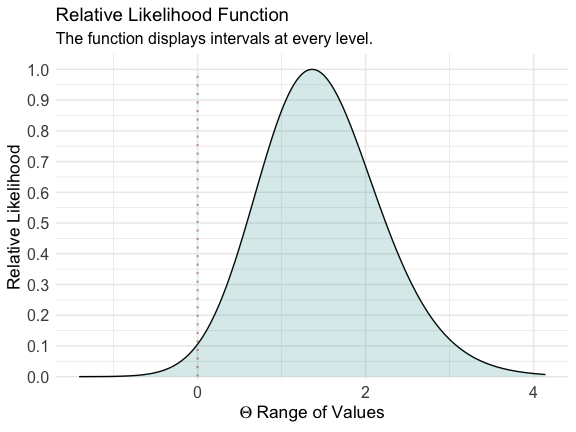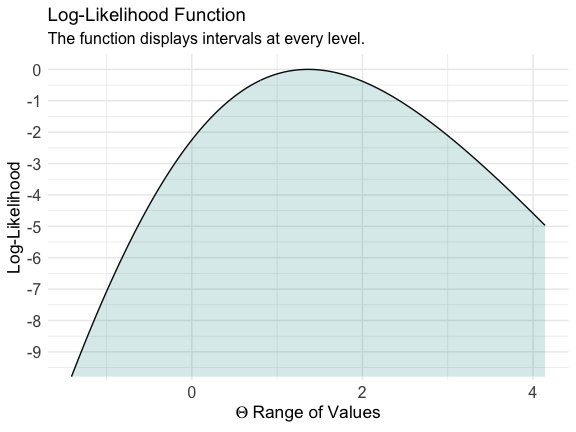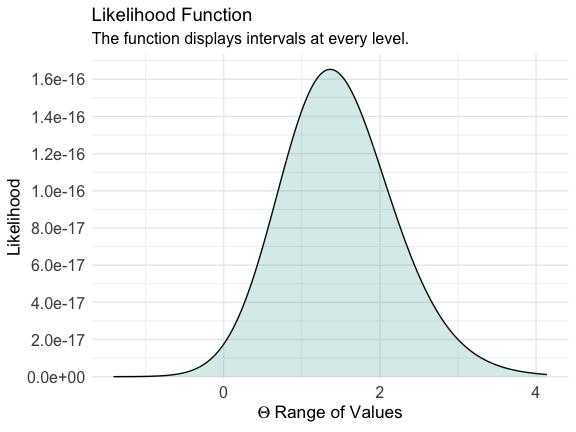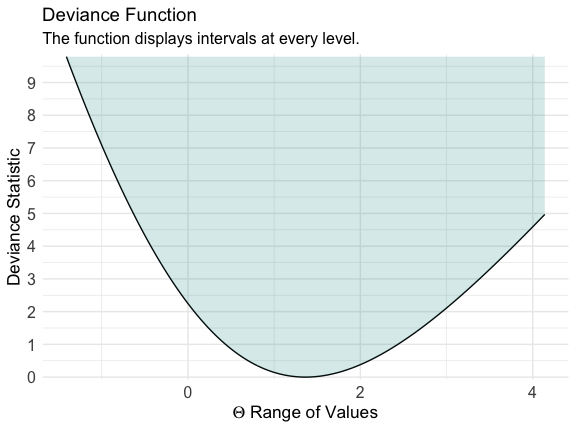# Profile Likelihoods

For this last example, we’ll explore the curve_lik() function, which can help generate profile likelihood functions, and deviance statistics with the help of the ProfileLikelihood package.1

library(ProfileLikelihood)
#> Loading required package: MASS

We’ll use a simple example taken directly from the ProfileLikelihood documentation where we’ll calculate the likelihoods from a glm model

data(dataglm)
xx <- profilelike.glm(y ~ x1 + x2,
data = dataglm, profile.theta = "group",
family = binomial(link = "logit"), length = 500, round = 2
)
#> Warning message: provide lo.theta and hi.theta

Then, we’ll use curve_lik() on the object that the ProfileLikelihood package created.

lik <- curve_lik(xx, dataglm)

Next, we’ll plot three functions, the relative likelihood, the log-likelihood, the likelihood, and the deviance function.

ggcurve(lik[], type = "l1", nullvalue = TRUE)ggcurve(lik[], type = "l2")ggcurve(lik[], type = "l3")ggcurve(lik[], type = "d")The obvious advantage of using reduced likelihoods is that they are free of nuisance parameters

$L_{t_{n}}(\theta)=f_{n}\left(F_{n}^{-1}\left(H_{p i v}(\theta)\right)\right)\left|\frac{\partial}{\partial t} \psi\left(t_{n}, \theta\right)\right|=h_{p i v}(\theta)\left|\frac{\partial}{\partial t} \psi(t, \theta)\right| /\left.\left|\frac{\partial}{\partial \theta} \psi(t, \theta)\right|\right|_{t=t_{n}}$ thus, giving summaries of the data that can be incorporated into combined analyses.